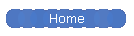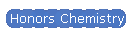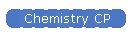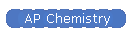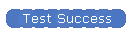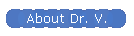The AP Chemistry exam is a three hour and fifteen minute timed exam, consisting of two sections.  Each section is weighted equally--i.e., each portion will determine 50% of the overall grade. Section 1--Multiple Choice  Students have 90 minutes to complete 60 multiple choice questions, which cover the entire curriculum.  Each multiple choice question will have 4 possible answers, and there is no "guessing penalty" for incorrect answers--the student score depends only on the number of questions answered correctly in this section.  Half of the multiple choice questions are presented as sets relating to a common data set or information.    Students are not allowed to use calculators during the multiple choice portion of the exam, but students will have access to a periodic table and an equations reference sheet.  Section 2--Free Response Students have 105 minutes to complete 7 open response items.  Experimental design and data analysis, as well as particle-level representations and computation problem solving,  will be distributed throughout this portion of the exam. Students complete three long response questions (about 20 minutes each) and four short response questions (about 7 minutes each).    The free response questions will include several parts and may cover a range of related topics.  Needless to say, answers should be brief and focused!  Calculators, including most graphing calculators,  may be used in this section.  Students may use the equations reference sheet and periodic table.     The AP chemistry exam will be the first AP exam given, usually the first Monday in May.  In 2016, the AP Chemistry exam will be given on Monday, May 2.